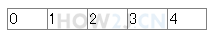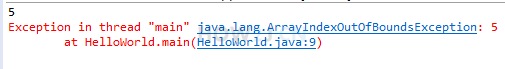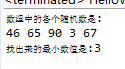8分35秒

## 声明数组

int[] a; 声明了一个数组变量。
[]表示该变量是一个数组
int 表示数组里的每一个元素都是一个整数
a 是变量名

public class HelloWorld { public static void main(String[] args) { // 声明一个数组 int[] a; } }
```public class HelloWorld {
public static void main(String[] args) {
// 声明一个数组
int[] a;
}
}
```

## 创建数组

new int

int c = 5; 这叫给c赋值为5

a = new int;public class HelloWorld { public static void main(String[] args) { //声明一个引用 int[] a; //创建一个长度是5的数组，并且使用引用a指向该数组 a = new int; int[] b = new int; //声明的同时，指向一个数组 } }
```public class HelloWorld {
public static void main(String[] args) {
//声明一个引用
int[] a;
//创建一个长度是5的数组，并且使用引用a指向该数组
a = new int;

int[] b = new int; //声明的同时，指向一个数组

}
}
```

## 访问数组public class HelloWorld { public static void main(String[] args) { int[] a; a = new int; a= 1; //下标0，代表数组里的第一个数 a= 2; a= 3; a= 4; a= 5; } }
```public class HelloWorld {
public static void main(String[] args) {
int[] a;
a = new int;

a= 1;  //下标0，代表数组里的第一个数
a= 2;
a= 3;
a= 4;
a= 5;
}
}
```

## 数组长度

.length属性用于访问一个数组的长度public class HelloWorld { public static void main(String[] args) { int[] a; a = new int; System.out.println(a.length); //打印数组的长度 a=100; //下标4，实质上是“第5个”，即最后一个 a=101; //下标5，实质上是“第6个”，超出范围 ,产生数组下标越界异常 } }
```public class HelloWorld {
public static void main(String[] args) {
int[] a;
a = new int;

System.out.println(a.length); //打印数组的长度

a=100; //下标4，实质上是“第5个”，即最后一个
a=101; //下标5，实质上是“第6个”，超出范围 ,产生数组下标越界异常

}
}
```

## 练习-数组最小值

姿势不对,事倍功半! 点击查看做练习的正确姿势

0-100的 随机整数的获取办法有多种，下面是参考办法之一:

(int) (Math.random() * 100)

Math.random() 会得到一个0-1之间的随机浮点数，然后乘以100，并强转为整型即可。
public class HelloWorld { public static void main(String[] args) { int[] a = new int; a = (int) (Math.random() * 100); a = (int) (Math.random() * 100); a = (int) (Math.random() * 100); a = (int) (Math.random() * 100); a = (int) (Math.random() * 100); System.out.println("数组中的各个随机数是:"); for (int i = 0; i < a.length; i++) System.out.println(a[i]); System.out.println("本练习的目的是，找出最小的一个值: "); } }
```public class HelloWorld {
public static void main(String[] args) {
int[] a = new int;
a = (int) (Math.random() * 100);
a = (int) (Math.random() * 100);
a = (int) (Math.random() * 100);
a = (int) (Math.random() * 100);
a = (int) (Math.random() * 100);

System.out.println("数组中的各个随机数是:");
for (int i = 0; i < a.length; i++)
System.out.println(a[i]);

System.out.println("本练习的目的是，找出最小的一个值: ");
}
}
```

## 答案-数组最小值3分49秒 本视频采用html5方式播放，如无法正常播放，请将浏览器升级至最新版本，推荐火狐，chrome，360浏览器。 如果装有迅雷，播放视频呈现直接下载状态，请调整 迅雷系统设置-基本设置-启动-监视全部浏览器 (去掉这个选项)。 chrome 的 视频下载插件会影响播放，如 IDM 等，请关闭或者切换其他浏览器public class HelloWorld { public static void main(String[] args) { int[] a = new int; a = (int) (Math.random() * 100); a = (int) (Math.random() * 100); a = (int) (Math.random() * 100); a = (int) (Math.random() * 100); a = (int) (Math.random() * 100); System.out.println("数组中的各个随机数是:"); for (int i = 0; i < a.length; i++) System.out.print(a[i] + " "); System.out.println(); int min = 100; for (int i = 0; i < a.length; i++) { if( a[i] < min ) min = a[i]; } System.out.println("找出来的最小数值是:" +min); } }

HOW2J公众号，关注后实时获知最新的教程和优惠活动，谢谢。2022-06-02 我写的
 wrj14 关于 JAVA 基础-数组-创建数组 的提问 我写的 ```public class findLeastArr { public static void main(String[] args) { int[] a=new int; a = (int)(Math.random() * 100); a = (int)(Math.random() * 100); a = (int)(Math.random() * 100); a = (int)(Math.random() * 100); a = (int)(Math.random() * 100); System.out.println("数组中的各个随机数是："); for (int i = 0; i < a.length; i++) { System.out.print(a[i]+"\t"); } int min=a; for (int i = 1; i < a.length; i++) { // int temp = a; min=(min < a[i])? min:a[i]; } System.out.println("最小值为："+ min); }```

5 个答案

lllfffzzz

rookie_star

import java.util.Arrays; public class getArrMinAndMaxNum { public static void main(String[] args) { int[] a = new int; System.out.println("数组中的随机数是："); for (int i = 0; i < a.length; i++) { a[i] = (int) (Math.random() * 100); System.out.println(a[i]); } //排序法 Arrays.sort(a); int minNum = a; int maxNum = a[a.length-1]; System.out.println("数组中最小的值是：" + minNum); System.out.println("数组中最大的值是：" + maxNum); //直接取值法 int max = Arrays.stream(a).max().getAsInt(); int min = Arrays.stream(a).min().getAsInt(); System.out.println("数组中最小的值是：" + min); System.out.println("数组中最大的值是：" + max); //遍历法 int mn = a; int mx = a; for (int i = 0; i < 5; i++) { if (a[i] < mn) mn = a[i]; } for (int i = 0; i < 5; i++) { if (a[i] > mx) mx =a [i]; } System.out.println("数组中最小的值是:"+mn); System.out.println("数组中最大的值是:"+mx); } }

rookie_star

import java.util.Arrays; public class ArrayMinNum { public static void main(String[] args) { int[] a = new int; System.out.println("数组中的随机数是："); for (int i = 0; i < a.length; i++) { a[i] = (int) (Math.random() * 100); System.out.println(a[i]); } Arrays.sort(a); int minNum = a; int maxNum = a[a.length-1]; System.out.println("数组中最小的值是：" + minNum); System.out.println("数组中最大的值是：" + maxNum); } }

javajava68

2022-03-18 如何简化成一个for loop
 frank417 关于 JAVA 基础-数组-创建数组 的提问 大神们我想用一个for loop 即打印数组所有的值又能找到最小值。 现在的话只能显示前四位。请问有什么办法吗 ```public class Getmin { public static void main(String[] args) { int[] a; int min=0; a = new int; int[] b = new int; a = (int) (Math.random() * 100); a = (int) (Math.random() * 100); a = (int) (Math.random() * 100); a = (int) (Math.random() * 100); a = (int) (Math.random() * 100); for (int i = 0; i < a.length-1; i++){ System.out.println(a[i]); if (a[i+1] < a[i]) min = a[i+1]; else min = a[i]; } System.out.println("min:"+min); } }```

7 个答案

bobtick

Java_Zheuser

public class ArrayTestMinValue { public static void main(String[] args) { int min_value =100; int max_value = 0; int [] array01 = new int; for (int i = 0; i < array01.length; i++) { array01[i] = (int) (Math.random() * 100); System.out.print(array01[i] + " "); if(array01[i] < min_value){ min_value = array01[i]; }else if(array01[i] >= max_value){ max_value = array01[i]; } } System.out.println(); System.out.println("随机数组中最小的元素是：" + min_value); System.out.println("随机数组中最大的元素是：" + max_value); } }

xiaosanye123

public class HelloWorld { public static void main(String[] args) { int s = 101; int[] a = new int; a = (int) (Math.random() * 100); a = (int) (Math.random() * 100); a = (int) (Math.random() * 100); a = (int) (Math.random() * 100); a = (int) (Math.random() * 100); System.out.println("数组中的各个随机数是:"); for (int i = 0; i < a.length; i++) { System.out.println(a[i]); if (a[i]<s){ s=a[i]; } } System.out.println("本练习的目的是，找出最小的一个值: "+s); } }

kunagisa

2021-11-16 数组最大值 最小值
2021-10-12 写方法写方法
2021-10-04 代码